# Discrete dynamical systems

### Discrete dynamical systems

#### Lessons

Assume that $A$ is diagonalizable, with $n$ linearly independent eigenvectors $v_1, v_2, \cdots , v_n$, and corresponding eigenvalues $\lambda _1, \lambda _2, \cdots \lambda _n$. Then we can write an initial vector $x_0$ to be:
$x_0=c_1 v_1+c_2 v_2+ \cdots +c_n v_n$

Let's say we want to transform $x_0$ with matrix $A$. Let's call the transformed vector to be $x_1$. Then,
$x_1=Ax_0=c_1 Av_1+c_2 Av_2+\cdots+c_n Av_n$
$=c_1 \lambda_1 v_1+c_2 \lambda_2 v_2+\cdots+c_n \lambda_n v_n$

Let's say we want to keep transforming it with matrix $A\; k$ times. Then we can generalize this to be:
$x_k=c_1 (\lambda_1 )^k v_1+c_2 (\lambda_2 )^k v_2+\cdots+c_n (\lambda_n )^k v_n$

This is useful because we get to know the behaviour of this equation when $k$$\infty$.
• Introduction
Discrete Dynamical Systems Overview:
a)
The Differential Equation $x_{k+1}=Ax_k$
• Linear Transformation
• Multiplying with A k times
• Generalized formula
• Why is this formula useful?

b)
Doing an Example
• Calculate $x_k$
• Long-term behaviour $(k$$\infty)$

c)
Application: Predator and Prey Model
• Analyzing the predator and prey equations
• Converting to the equation $x_{k+1}=Ax_k$
• Calculating the general solution
• Long term behaviour $(k$$\infty)$

• 1.
Finding the General Solution
Let $A$ be a $3 \times 3$ matrix with eigenvalues $3,2,$ and $\frac{1}{2}$, and corresponding eigenvectors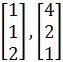and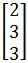. if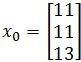, find the general solution of the equation $x_{k+1}=Ax_k$.

• 2.
Analyzing the Long Term Behaviour
Explain the long term behaviour $(k$$\infty)$ of the equation $x_{k+1}=Ax_k$, where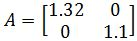And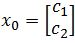where $c_1$ > $0$ and $c_2$ > $0$.

• 3.
Explain the long term behaviour $(k$$\infty)$ of the equation $x_{k+1}=Ax_k$, where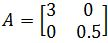Andwhere $c_1$ > $0$ and $c_2$ > $0$

• 4.
Predator and Prey Model
Let the eagle and rabbit population at time $k$ be denoted as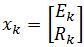, where $k$ is the time in years, $E_k$ is the number of eagles at time $k$, and $R_k$ is the number of rabbits at time $k$ (all measured in thousands). Suppose there are two equations describing the relationship between these two species:

$E_{k+1}=(.4) E_k+(.5)R_k$
$R_{k+1}=(-.207) E_k+(1.2) R_k$
a)
What happens to the rabbits if there are no eagles? What happens to the eagles if there are no rabbits?

b)
Find the matrix $A$ for the equation $x_{k+1}=Ax_k$.

c)
Suppose the eigenvalues of $A$ is $\lambda_1=1.0377$ and $\lambda_2=0.562303$ and the corresponding eigenvectors are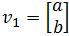and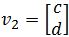(assume $a,b,c,d$ > $0$). Find the general solution.

d)
Assuming that $c_1,\;c_2$ > $0$, explain the population of rabbits and eagles as $k$$\infty$.